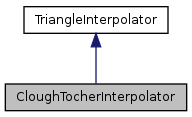QGIS API Documentation  2.6.0-Brighton
CloughTocherInterpolator Class Reference

This is an implementation of a Clough-Tocher interpolator based on a triangular tessellation. More...

`#include <CloughTocherInterpolator.h>`

Inheritance diagram for CloughTocherInterpolator:[legend]

## Public Member Functions

CloughTocherInterpolator ()
standard constructor
CloughTocherInterpolator (NormVecDecorator *tin)
constructor with a pointer to the triangulation as argument
virtual ~CloughTocherInterpolator ()
destructor
virtual bool calcNormVec (double x, double y, Vector3D *result)
Calculates the normal vector and assigns it to vec (not implemented at the moment)
virtual bool calcPoint (double x, double y, Point3D *result)
Performs a linear interpolation in a triangle and assigns the x-,y- and z-coordinates to point.
virtual void setTriangulation (NormVecDecorator *tin)Public Member Functions inherited from TriangleInterpolator
virtual ~TriangleInterpolator ()

## Protected Member Functions

void init (double x, double y)
Finds out, in which triangle the point with the coordinates x and y is.
double calcBernsteinPoly (int n, int i, int j, int k, double u, double v, double w)
Calculates the Bernsteinpolynomials to calculate the Beziertriangle.

## Protected Attributes

NormVecDecoratormTIN
association with a triangulation object
double mEdgeTolerance
Tolerance of the barycentric coordinates at the borders of the triangles (to prevent errors because of very small negativ baricentric coordinates)
Point3D point1
first point of the triangle in x-,y-,z-coordinates
Point3D point2
second point of the triangle in x-,y-,z-coordinates
Point3D point3
third point of the triangle in x-,y-,z-coordinates
Point3D cp1
Point3D cp2
Point3D cp3
Point3D cp4
Point3D cp5
Point3D cp6
Point3D cp7
Point3D cp8
Point3D cp9
Point3D cp10
Point3D cp11
Point3D cp12
Point3D cp13
Point3D cp14
Point3D cp15
Point3D cp16
double der1X
derivative in x-direction at point1
double der1Y
derivative in y-direction at point1
double der2X
derivative in x-direction at point2
double der2Y
derivative in y-direction at point2
double der3X
derivative in x-direction at point3
double der3Y
derivative in y-direction at point3
Point3D lpoint1
stores point1 of the last run
Point3D lpoint2
stores point2 of the last run
Point3D lpoint3
stores point3 of the last run

## Detailed Description

This is an implementation of a Clough-Tocher interpolator based on a triangular tessellation.

The derivatives orthogonal to the boundary curves are interpolated linearly along a triangle edge.

## Constructor & Destructor Documentation

 CloughTocherInterpolator::CloughTocherInterpolator ( )
inline

standard constructor

 CloughTocherInterpolator::CloughTocherInterpolator ( NormVecDecorator * tin )
inline

constructor with a pointer to the triangulation as argument

 CloughTocherInterpolator::~CloughTocherInterpolator ( )
inlinevirtual

destructor

## Member Function Documentation

 double CloughTocherInterpolator::calcBernsteinPoly ( int n, int i, int j, int k, double u, double v, double w )
protected

Calculates the Bernsteinpolynomials to calculate the Beziertriangle.

'n' is three in the cubical case, 'i', 'j', 'k' are the indices of the controllpoint and 'u', 'v', 'w' are the barycentric coordinates of the point

 virtual bool CloughTocherInterpolator::calcNormVec ( double x, double y, Vector3D * result )
virtual

Calculates the normal vector and assigns it to vec (not implemented at the moment)

Implements TriangleInterpolator.

 virtual bool CloughTocherInterpolator::calcPoint ( double x, double y, Point3D * result )
virtual

Performs a linear interpolation in a triangle and assigns the x-,y- and z-coordinates to point.

Implements TriangleInterpolator.

 void CloughTocherInterpolator::init ( double x, double y )
protected

Finds out, in which triangle the point with the coordinates x and y is.

 void CloughTocherInterpolator::setTriangulation ( NormVecDecorator * tin )
inlinevirtual

## Member Data Documentation

 Point3D CloughTocherInterpolator::cp1
protected
 Point3D CloughTocherInterpolator::cp10
protected
 Point3D CloughTocherInterpolator::cp11
protected
 Point3D CloughTocherInterpolator::cp12
protected
 Point3D CloughTocherInterpolator::cp13
protected
 Point3D CloughTocherInterpolator::cp14
protected
 Point3D CloughTocherInterpolator::cp15
protected
 Point3D CloughTocherInterpolator::cp16
protected
 Point3D CloughTocherInterpolator::cp2
protected
 Point3D CloughTocherInterpolator::cp3
protected
 Point3D CloughTocherInterpolator::cp4
protected
 Point3D CloughTocherInterpolator::cp5
protected
 Point3D CloughTocherInterpolator::cp6
protected
 Point3D CloughTocherInterpolator::cp7
protected
 Point3D CloughTocherInterpolator::cp8
protected
 Point3D CloughTocherInterpolator::cp9
protected
 double CloughTocherInterpolator::der1X
protected

derivative in x-direction at point1

 double CloughTocherInterpolator::der1Y
protected

derivative in y-direction at point1

 double CloughTocherInterpolator::der2X
protected

derivative in x-direction at point2

 double CloughTocherInterpolator::der2Y
protected

derivative in y-direction at point2

 double CloughTocherInterpolator::der3X
protected

derivative in x-direction at point3

 double CloughTocherInterpolator::der3Y
protected

derivative in y-direction at point3

 Point3D CloughTocherInterpolator::lpoint1
protected

stores point1 of the last run

 Point3D CloughTocherInterpolator::lpoint2
protected

stores point2 of the last run

 Point3D CloughTocherInterpolator::lpoint3
protected

stores point3 of the last run

 double CloughTocherInterpolator::mEdgeTolerance
protected

Tolerance of the barycentric coordinates at the borders of the triangles (to prevent errors because of very small negativ baricentric coordinates)

 NormVecDecorator* CloughTocherInterpolator::mTIN
protected

association with a triangulation object

 Point3D CloughTocherInterpolator::point1
protected

first point of the triangle in x-,y-,z-coordinates

 Point3D CloughTocherInterpolator::point2
protected

second point of the triangle in x-,y-,z-coordinates

 Point3D CloughTocherInterpolator::point3
protected

third point of the triangle in x-,y-,z-coordinates

The documentation for this class was generated from the following file: